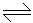# Chemical Engineering - Chemical Reaction Engineering

### Exercise :: Chemical Reaction Engineering - Section 2

36.

The effect of increasing pressure on the gaseous equilibrium of the reaction 2X+3Y3X+2Y indicates that

 A. pressure has no effect. B. backward reaction is favoured. C. forward reaction is favoured. D. none of these.

Explanation:

No answer description available for this question. Let us discuss.

37.

If in the gaseous phase reaction, N2O42NO2, x is the part of N2O4 which dissociates, then the number of molecules at equilibrium will be

 A. (1 + x) B. (1 - x) C. (1 + x)2 D. (1 - x)2

Explanation:

No answer description available for this question. Let us discuss.

38.

A batch reactor is characterised by

 A. constant residence time. B. variation in extent of reaction and properties of the reaction mixture with time. C. variation in reactor volume. D. very low conversion.

Explanation:

No answer description available for this question. Let us discuss.

39.

When a catalyst increases the rate of forward reaction, the value of rate constant

 A. increases B. decreases C. remain same D. becomes infinite

Explanation:

No answer description available for this question. Let us discuss.

40.

Rate determining step in a reaction consisting of a number of steps in series is the __________ step.

 A. fastest B. slowest C. intermediate D. data insufficient; can't be predicted